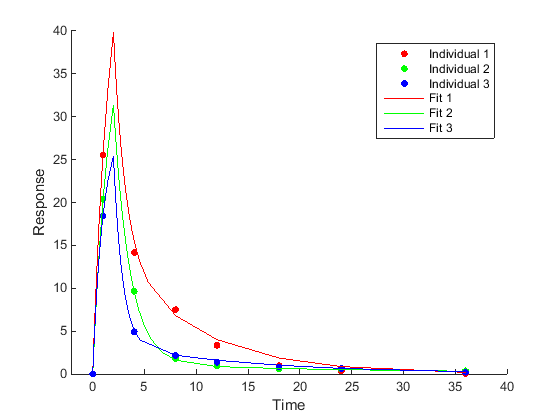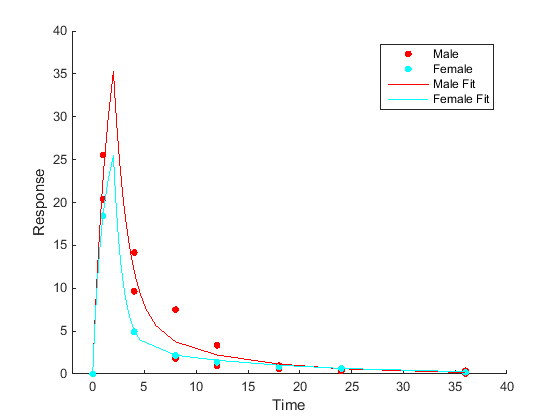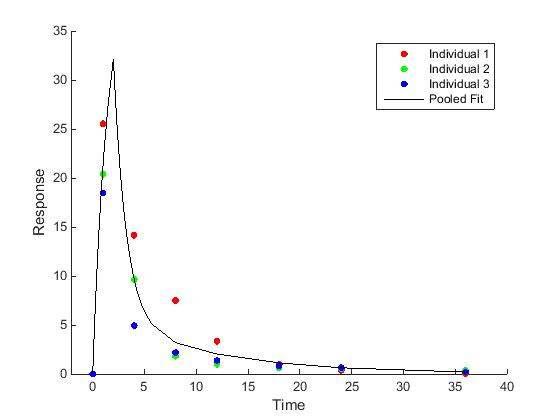## Nonlinear Regression

### What is Nonlinear Regression?

The purpose of regression models is to describe a response variable as a function of independent variables. Multiple linear regression models describe the response as a linear combination of coefficients and functions of independent variables. Nonlinearities can be modeled using nonlinear functions of independent variables. However, the coefficients always enter the model in a linear fashion.

Nonlinear regression models are more mechanistic models of nonlinear relationships between the response and independent variables. The parameters can enter the model as exponential, trigonometric, power, or any other nonlinear function. The unknown parameters in the model are estimated by minimizing a statistical criterion such as the negative log likelihood or the sum of squared deviations between observed and predicted values.

In the case of pharmacokinetic (PK) studies, the response data usually represent some measured drug concentrations, and independent variables are often dose and time. The nonlinear function often used for such data is an exponential function since many drugs once distributed in a patient are eliminated in an exponential fashion. One PK parameter to estimate in this case is the rate at which the drug is eliminated from the body given the concentration-time data.

For instance, consider drug plasma concentration data from a single individual after an intravenous bolus dose measured at different time points with some errors. Assume the measured drug concentration follows a monoexponential decline: ${C}_{t}={C}_{0}{e}^{-{k}_{e}t}+a\epsilon$.

This model describes the time course of drug concentration in the body (Ct), as a function of the drug concentration after an intravenous bolus dose at t = 0 (C0), time (t), and elimination rate parameter (ke). ε is the mean-zero and unit-variance variable, that is, $\epsilon \sim N\left(0,1\right)$ representing the measurement error and a is the error model parameter (here, standard deviation).

More generically, you can write the model as ${y}_{j}=f\left({x}_{j};p\right)+g\left({\epsilon }_{j}\right)$, where yj is the jth response of interest (such as Ct), f is a function of known quantities x (such as C0 and time t), model parameters p (such as ke), and an error model $g\left({\epsilon }_{j}\right)$.

If there are multiple observations on multiple individuals, the model becomes ${y}_{ij}=f\left({x}_{ij};{p}_{j}\right)\text{\hspace{0.17em}}+g\left({\epsilon }_{ij}\right)$ where yij is the jth observation of the ith individual. Additionally, you can categorize your data into different groups based on different categories such as sex, age, or height.

### Fitting Options in SimBiology

This table summarizes nonlinear regression options available in SimBiology®.

 Fitting Option Example Individual-specific parameter estimation (Unpooled fitting)Fit each individual separately, resulting in one set of parameter estimates for each individual.Category- or group-specific parameter estimationFit each category or group separately, resulting in one set of parameter estimates for each category.Population-wide parameter estimation (Pooled fitting)Fit all of the data pooled together, resulting in just one set of parameter estimates.In addition, SimBiology supports four kinds of error models for measured or observed responses, namely, constant (default), proportional, combined, and exponential. For details, see Error Models. Depending on the optimization method, you can specify an error model for each response or all responses. For details, see Supported Methods for Parameter Estimation in SimBiology.

### Maximum Likelihood Estimation

SimBiology estimates parameters by the method of maximum likelihood. Rather than directly maximizing the likelihood function, SimBiology constructs an equivalent minimization problem. Whenever possible, the estimation is formulated as a weighted least squares (WLS) optimization that minimizes the sum of the squares of weighted residuals. Otherwise, the estimation is formulated as the minimization of the negative of the logarithm of the likelihood (NLL). The WLS formulation often converges better than the NLL formulation, and SimBiology can take advantage of specialized WLS algorithms, such as the Levenberg-Marquardt algorithm implemented in lsqnonlin and lsqcurvefit. SimBiology uses WLS when there is a single error model that is constant, proportional, or exponential. SimBiology uses NLL if you have a combined error model or a multiple-error model, that is, a model having an error model for each response.

`sbiofit` supports different optimization methods, and passes in the formulated WLS or NLL expression to the optimization method that minimizes it.

Expression that is being minimized
Weighted Least Squares (WLS)For the constant error model, $\sum _{i}^{N}{\left({y}_{i}-{f}_{i}\right)}^{2}$
For the proportional error model, $\sum _{i}^{N}\frac{{\left({y}_{i}-{f}_{i}\right)}^{2}}{{f}_{i}^{2}/{f}_{gm}^{2}}$
For the exponential error model, $\sum _{i}^{N}{\left(\mathrm{ln}{y}_{i}-\mathrm{ln}{f}_{i}\right)}^{2}$
For numeric weights, $\sum _{i}^{N}\frac{{\left({y}_{i}-{f}_{i}\right)}^{2}}{{w}_{gm}/{w}_{i}}$
Negative Log-likelihood (NLL)For the combined error model and multiple-error model, $\sum _{i}^{N}\frac{{\left({y}_{i}-{f}_{i}\right)}^{2}}{2{\sigma }_{i}^{2}}+\sum _{i}^{N}\mathrm{ln}\sqrt{2\pi {\sigma }_{i}^{2}}$

The variables are defined as follows.

 N Number of experimental observations yi The ith experimental observation ${f}_{i}$ Predicted value of the ith observation ${\sigma }_{i}$ Standard deviation of the ith observation.For the constant error model, ${\sigma }_{i}=a$For the proportional error model, ${\sigma }_{i}=b|{f}_{i}|$For the combined error model, ${\sigma }_{i}=a+b|{f}_{i}|$ ${f}_{gm}$ ${f}_{gm}={\left(\prod _{i}^{N}|{f}_{i}|\right)}^{1}{N}}$ ${w}_{i}$ The weight of the ith predicted value ${w}_{gm}$ ${w}_{gm}={\left(\prod _{i}^{N}{w}_{i}\right)}^{1}{N}}$

When you use numeric weights or the weight function, the weights are assumed to be inversely proportional to the variance of the error, i.e., ${\sigma }_{i}^{2}=\frac{{a}^{2}}{{w}_{i}}$ where a is the constant error parameter. If you use weights, you cannot specify an error model except the constant error model.

Various optimization methods have different requirements on the function that is being minimized. For some methods, the estimation of model parameters is performed independently of the estimation of the error model parameters. The following table summarizes the error models and any separate formulas used for the estimation of error model parameters, where a and b are error model parameters and e is the standard mean-zero and unit-variance (Gaussian) variable.

Error ModelError Parameter Estimation Function
`'constant'`: ${y}_{i}={f}_{i}+ae$${a}^{2}=\frac{1}{N}\sum _{i}^{N}{\left({y}_{i}-{f}_{i}\right)}^{2}$
`'exponential'`: ${y}_{i}={f}_{i}\mathrm{exp}\left(ae\right)$${a}^{2}=\frac{1}{N}\sum _{i}^{N}{\left(\mathrm{ln}{y}_{i}-\mathrm{ln}{f}_{i}\right)}^{2}$
`'proportional'`: ${y}_{i}={f}_{i}+b|{f}_{i}|e$${b}^{2}=\frac{1}{N}\sum _{i}^{N}{\left(\frac{{y}_{i}-{f}_{i}}{{f}_{i}}\right)}^{2}$
`'combined'`: ${y}_{i}={f}_{i}+\left(a+b|{f}_{i}|\right)e$Error parameters are included in the minimization.
Weights${a}^{2}=\frac{1}{N}\sum _{i}^{N}{\left({y}_{i}-{f}_{i}\right)}^{2}{w}_{i}$

### Note

`nlinfit` only support single error models, not multiple-error models, that is, response-specific error models. For a combined error model, it uses an iterative WLS algorithm. For other error models, it uses the WLS algorithm as described previously. For details, see `nlinfit`.

### Fitting Workflow for sbiofit

The following steps show one of the workflows you can use at the command line to fit a PK model.

1. Convert the data to the `groupedData` format.

2. Define dosing data. For details, see Doses in SimBiology Models.

3. Create a structural model (one-, two-, or a multicompartment model). For details, see Create Pharmacokinetic Models.

4. Map the response variable from data to the model component. For example, if you have the measured drug concentration data for the central compartment, then map it to the drug species in the central compartment (typically the `Drug_Central` species).

5. Specify parameters to estimate using an `EstimatedInfo object`. Optionally, you can specify parameter transformations, initial values, and parameter bounds.

6. Perform parameter estimation using `sbiofit`.

For illustrated examples, see the following.